# The Deca-augmented 5,10-duoprism

The deca-augmented 5,10-duoprism is a CRF polychoron bounded by 5 decagonal prisms, 50 square pyramids, and 10 pentagonal bipyramids (J13) for a total of 65 cells, 205 polygons (5 decagons, 50 squares, and 150 triangles), 200 edges, and 60 vertices.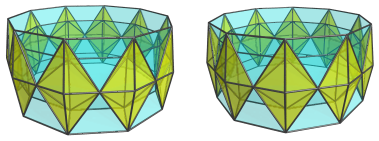It is constructed by augmenting the 5,10-duoprism with 10 pentagonal prism pyramids. Due to the dichoral angle between the pentagonal prisms in the 5,10-duoprism being exactly 144°, and the dichoral angle between the pentagonal pyramids and pentagonal prism of the pentagonal prism pyramid being exactly 18°, the pentagonal pyramid cells of adjacent augments lie on the same hyperplanes, thus merging into 10 pentagonal bipyramids (J13).

It can be partially Stott-expanded along the symmetries of the decagons to yield the deca-augmented 5,20-duoprism, which has the same structure except with elongated pentagonal bipyramids (J16) instead of the J13 cells, and icosagonal prisms instead of the decagonal prisms.

## Structure

### Centered on Ring of Decagonal Prisms

The deca-augmented 5,10-duoprism consists of a ring of 5 decagonal prisms: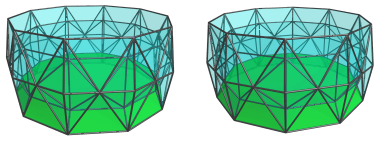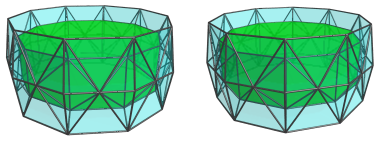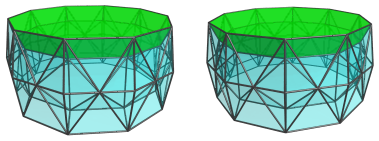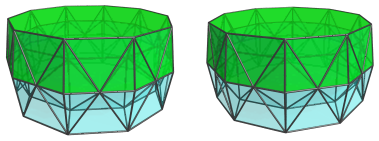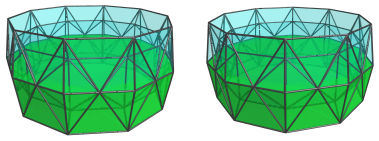This ring of cells intertwines with an orthogonal ring of pentagonal bipyramids, strung to each other by their apical vertices: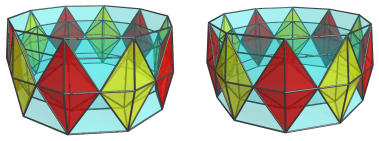These pentagonal bipyramids are straddled by square pyramids, 5 surrounding each shared apex of the pentagonal bipyramids, for 50 square pyramids in total: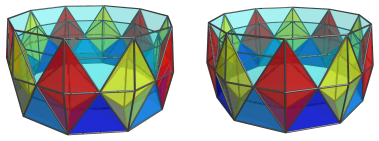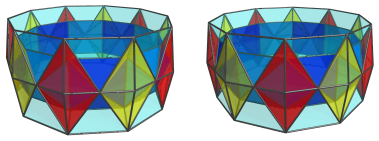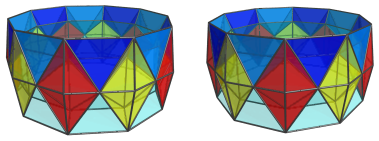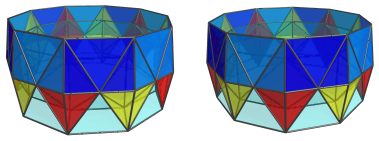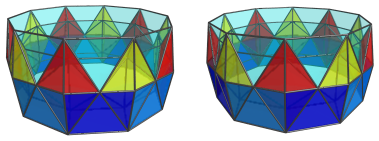These are all the cells of the deca-augmented 5,10-duoprism.

### Centered on a Pentagonal Bipyramid

#### The Near Side

Now we look at the deca-augmented 5,10-duoprism from a different 4D viewpoint, one that allows us to see the J13 cells more clearly.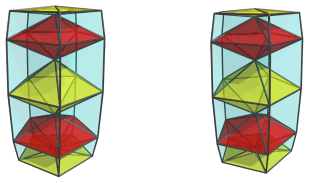The above image shows 5 of the pentagonal bipyramid (J13) cells that face this new 4D viewpoint.

These J13 cells are straddled by 20 square pyramids: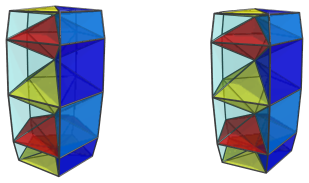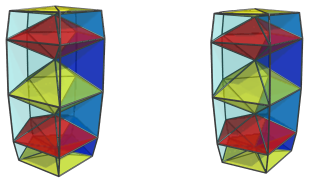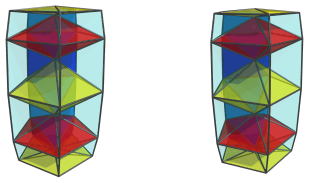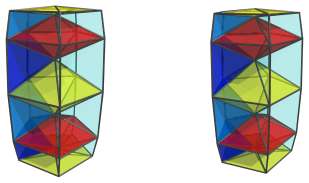These are all the cells that lie on the near side of the polychoron.

#### The Far Side

Next, we come to the far side of the polytope. Here are the other 5 pentagonal bipyramids: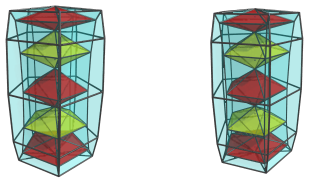There are 30 square pyramids straddling these cells: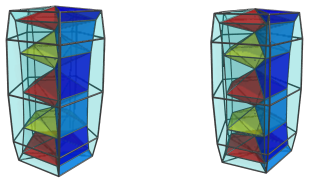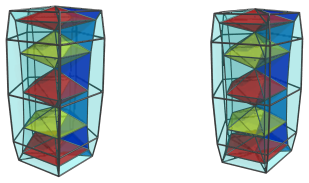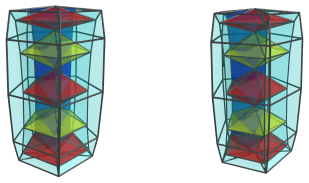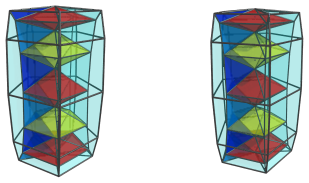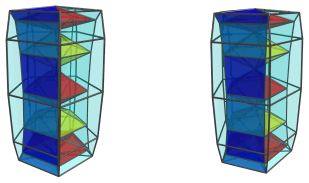Finally, the square faces of all the square pyramids we've seen so far, including those on the near side of the polychoron, are where the 5 decagonal prisms are fitted: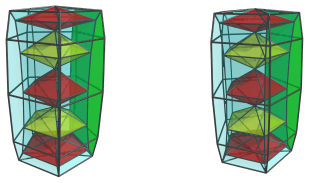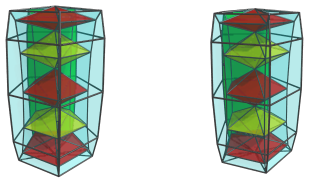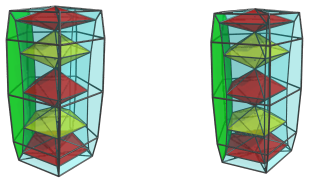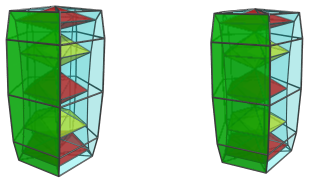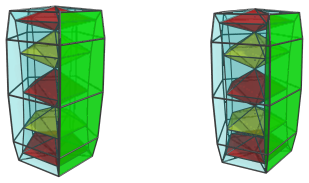These decagonal prisms lie at a steep angle from the 4D viewpoint, and therefore appear quite squished by foreshortening. They are, of course, perfectly uniform in 4D.

#### Summary

The following table summarizes the cell counts:

Region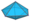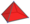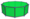Near side 5 20 -
Far side 5 30 5
Grand total 10 50 5
65 cells

## Coordinates

The Cartesian coordinates of the deca-augmented 5,10-duoprism, centered on the origin and with edge length 2, are:

• (A, 0, ±2φ, 0)
• (B, ±φ, ±2φ, 0)
• (−C, ±1, ±2φ, 0)
• (A, 0, ±1, ±√(3+4φ))
• (B, ±φ, ±1, ±√(3+4φ))
• (−C, ±φ, ±1, ±√(3+4φ))
• (A, 0, φ2, ±√(2+φ))
• (B, ±φ, φ2, ±√(2+φ))
• (−C, ±φ, φ2, ±√(2+φ))
• (0, 0, 0, ±2A)
• (0, 0, ±2φ, ±2B)
• (0, 0, ±2, ±2C)

where:

 A = √((10+2√5)/5) B = √((5−√5)/10) C = √((5+2√5)/5)

and φ=(1+√5)/2 is the Golden Ratio.

Last updated 01 May 2019.